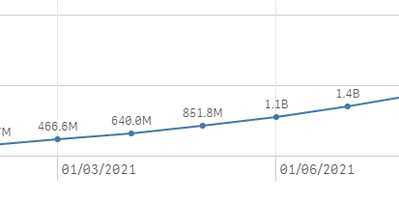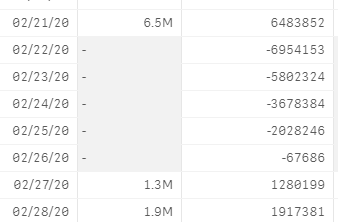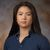# New to Qlik Sense

If you’re new to Qlik Sense, start with this Discussion Board and get up-to-speed quickly.

Announcements
Qlik Cloud Maintenance is scheduled between March 27-30. Visit Qlik Cloud Status page for more details.
cancel
Showing results for
Did you mean:Contributor III

## Number Formatting issue while changing billions/Millions in trend line chart

Hi Guys..

1.Do we have  any options to set 1 decimal point  while using number format  as 'AUTO' ,by default qliksense displaying 2 decimal points in auto format.

to achieve this  i have done below logic

1. set  as single decimal point   2. to convert my numbers to  Millions(#M),Billions(#B),Thousands(#k)..

But When i use trend line chart  with same logic.. chart not displaying on unit type.  trendline showing only based on value.. (due to hard coded..)

=if( \$(Value1)<1000000, Num(\$(Value1)/1000,'#.0'&'K)'), if( \$(Value1)<1000000000 , Num(\$(Value1)/1000000,'#.0MM'),
Num(\$(Value1)/1000000000,'#.0B')))

Choosing Number format as "measure Expression"

Trend Chart  below showing  4.5MM to 640.0 k  going up arrow instead of downfall..  is there any way to  display proper line..@Sunny@qlik

Labels (5)

• ### SaaS

1 Solution

Accepted SolutionsPartner - Creator

Hi there!

That was a tricky challenge! Please find a fully worked example of how you can solve it attached.

I'd encourage you to study the example file, but if you are short of time and just need the syntax - here is the measure expression I used - just substitute your own expression for every instance of sum(Value)

dual(num(sum(Value)/pow(10,3*floor(log10(sum(Value))/3)),'#,###.#'&pick(floor(log10(sum(Value))/3),'K','M','B')),sum(Value))

It leverages the dual() function to retain the original expression as the numerical value whilst manipulating the text representation tag, which is what is displayed on each data point. Screen below shows example output.Best regards

-Jonas

16 RepliesPartner - Creator

Hi there!

That was a tricky challenge! Please find a fully worked example of how you can solve it attached.

I'd encourage you to study the example file, but if you are short of time and just need the syntax - here is the measure expression I used - just substitute your own expression for every instance of sum(Value)

dual(num(sum(Value)/pow(10,3*floor(log10(sum(Value))/3)),'#,###.#'&pick(floor(log10(sum(Value))/3),'K','M','B')),sum(Value))

It leverages the dual() function to retain the original expression as the numerical value whilst manipulating the text representation tag, which is what is displayed on each data point. Screen below shows example output.Best regards

-JonasContributor III
Author

Thank you so much.. Jonus

it helps a lot..   much appreciated for quick responseContributor III
Author

how can make it work for   negative values(-)   ?

dual(num(sum(Value)/pow(10,3*floor(log10(sum(Value))/3)),'#,###.#'&pick(floor(log10(sum(Value))/3),'K','M','B')),sum(Value))

currently its not showing negative values in my trend line chart,,  Showing empty whenever negative values comingContributor
Set vFormatNum = Dual(Num(\$1/ Pow(10, 3 * Div(Log10(fabs(\$1)),3)),'\$#,##0.0'& ' ' & Pick(Div(Log10(fabs(\$1)), 3),'K','M','B','T'), \$1)

In Measure Expression Editor
\$(vFormatNum({insert expression here})

Set Number Formatting to "Measure Expression" if needed.MVP

@kdr_Qv  use dual function to represent actual values on axis like below

=dual(if( \$(Value1)<1000000, Num(\$(Value1)/1000,'#.0'&'K)'), if( \$(Value1)<1000000000 , Num(\$(Value1)/1000000,'#.0MM'),
Num(\$(Value1)/1000000000,'#.0B'))),\$(Value1))MVP

@kdr_Qv  further you can use pick match to simplify expression like below

dual (pick(match(-1, \$(Value1)>=1000000000,\$(Value1)>=1000000)+1,

num(\$(Value1)/1000,'#,##0.0K')
num(\$(Value1)/1000000000,'#,##0.0B'),
num(\$(Value1)/1000000,'#,##0.0M'))
,\$(Value1))Contributor III
Author

Thank you @Kushal_ChawdaContributor III
Author

Thank you  @tonbaoMVP

Hi ,

you can try like this.

create inline in script.

Data_Format:
Format,FormatId
\$-Million,1000000
\$-Thousands,1000
];

front end exp:

vFormatId= FormatId

sum(Value)/\$(vFormatId)Tags
Community Browser